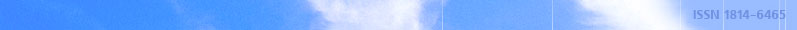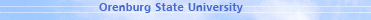december 03, 2021 RU/EN
 Headings of Vestnik Pedagogics Psychology Other

 Search Author Article

# 2010, № 9

Pushkov S.G., Gorelik A.A. THE USE OF METHODS OF INTERVAL ANALYSIS FOR ENUMERATING THE DIMENSIONALITY OF THE FINITE-DIMENSIONAL REALIZATION OF LINEAR DYNAMIC SYSTEMThe article examined the task of constructing the model in the state space on the basis of the data about the behavior the input-output of linear stationary dynamic system with the discrete time. The authors investigated the problem of obtaining the numerical algorithms of the calculation of finite-dimensional realization in the state space of mapping input-output, represented by the pulse sequence of matrices. They proposed the method of enumerating the dimensionality of this realization, based on the use of interval analysis. Key words: dynamic systems, state space, the problem of realization, numerical methods, interval analysis.

References:

1. Kalman, R. Essays on the mathematical theory of systems / R. Kalman, P. Falb, MA Arbib. — M.: Editorial URSS, 2004. — 400 pp.

2. Pushkov, SG On the computation of finite realization / SG GUN / / Cybernetics and system analysis. — 1991 № 6. — S. 107-112.

3. Pushkov, SG Representation of dynamic systems in state space: an exact and approximate realization / SG Pushkov. — Barnaul: Altai State Technical University Publishing House, 2003. — 270 pp.

4. Kahaner, D. Numerical methods and software / D. Kahaner, C. Mowler, C. Nash. -M.: Mir, 1998. — 575 pp., Il.

5. Alefeld, G. Introduction to interval computation / G. Alefeld, J. Hertsberger. — M.: Mir, 1987. — 360 pp.Editor-in-chief Sergey Aleksandrovich MIROSHNIKOV